# 1.1 -Basics of geological modeling with GemPy¶

Importing GemPy

import gempy as gp

# Importing auxiliary libraries
import numpy as np
import pandas as pd
import matplotlib.pyplot as plt
import os

# Setting options
np.random.seed(1515)
pd.set_option('precision', 2)


## Importing and creating a set of input data¶

The data used for the construction of a model in GemPy is stored in Python objects. The main data classes are:

-  Surface_points
-  Orientations
-  Grid
-  Surfaces
-  Series
-  Faults


We will see each of this class in further detail in the future.

Most of data can also be generated from raw data that comes in the form of CSV-files (CSV = comma-separated values). Such files might be attained by exporting model data from a different program such as GeoModeller or by simply creating it in spreadsheet software such as Microsoft Excel or LibreOffice Calc.

In this tutorial, all input data is created by importing such CSV-files. These exemplary files can be found in the input_data folder in the root folder of GemPy. The data comprises $$x$$-, $$y$$- and $$z$$-positional values for all surface points and orientation measurements. For the latter, poles, azimuth and polarity are additionally included. Surface points are furthermore assigned a formation. This might be a lithological unit such as “Sandstone” or a structural feature such as “Main Fault”. It is decisive to remember that, in GemPy, interface position points mark the bottom of a layer. If such points are needed to resemble a top of a formation (e.g. when modeling an intrusion), this can be achieved by defining a respectively inverted orientation measurement.

As we generate our Data from CSV-files, we also have to define our model’s real extent in $$x$$, $$y$$ and $$z$$, as well as declare a desired resolution for each axis. This resolution will in turn determine the number of voxels used during modeling. Here, we rely on a medium resolution of 50x50x50, amounting to 125,000 voxels. The model extent should be chosen in a way that it contains all relevant data in a representative space. As our model voxels are not cubes, but prisms, the resolution can take a different shape than the extent. We don’t recommend going much higher than 100 cells in every direction (1,000,000 voxels), as higher resolutions will become increasingly expensive to compute.

geo_model = gp.create_model('Tutorial_ch1_1_Basics')

data_path = 'https://raw.githubusercontent.com/cgre-aachen/gempy_data/master/'
# Importing the data from CSV-files and setting extent and resolution
gp.init_data(geo_model, [0, 2000., 0, 2000., 0, 750.], [50, 50, 50],
path_o=data_path + "/data/input_data/getting_started/"
"simple_fault_model_orientations.csv",
path_i=data_path + "/data/input_data/getting_started/"
"simple_fault_model_points.csv",
default_values=True)


Out:

Active grids: ['regular']

Tutorial_ch1_1_Basics  2021-04-18 11:28

geo_model.surfaces

surface series order_surfaces color id
0 Shale Default series 1 #015482 1
1 Sandstone_1 Default series 2 #9f0052 2
2 Siltstone Default series 3 #ffbe00 3
3 Sandstone_2 Default series 4 #728f02 4
4 Main_Fault Default series 5 #443988 5
5 basement Basement 1 #ff3f20 6

The input data can then be listed using the command get_data. Note that the order of formations and respective allocation to series is still completely arbitrary. We will fix this in the following.

gp.get_data(geo_model, 'surface_points').head()

X Y Z smooth surface
0 1000 50 450.00 2.00e-06 Shale
1 1000 150 433.33 2.00e-06 Shale
2 1000 300 433.33 2.00e-06 Shale
3 1000 500 466.67 2.00e-06 Shale
4 1000 1000 533.33 2.00e-06 Shale

gp.get_data(geo_model, 'orientations').head()

X Y Z G_x G_y G_z smooth surface
0 1000 1000 300 0.32 1.00e-12 0.95 0.01 Shale
1 400 1000 420 0.32 1.00e-12 0.95 0.01 Sandstone_2
2 500 1000 300 -0.95 1.00e-12 0.32 0.01 Main_Fault

### Declaring the sequential order of geological formations¶

• TODO update this section

We want our geological units to appear in the correct order relative to age. Such order might for example be given by a depositional sequence of stratigraphy, unconformities due to erosion or other lithological genesis events such as igneous intrusions. A similar age-related order is to be declared for the faults in our model. In GemPy, the function set_series is used to assign formations to different sequential series via declaration in a Python dictionary.

Defining the correct order of series is vital to the construction of the model! If you are using Python >3.6, the age-related order will already be defined by the order of key entries, i.e. the first entry is the youngest series, the last one the oldest. For older versions of Python, you will have to specify the correct order as a separate list attribute “order_series” (see cell below).

You can assign several surfaces to one series. The order of the units within such as series is only relevant for the color code, thus we recommend to be consistent. You can define this order via another attribute “order_formations/ ” or by using the specific command set_order_formations. (If the order of the pile differs from the final result the color of the interfaces and input data will be different.)

Every fault is treated as an independent series and have to be at set at the top of the pile. The relative order between the distinct faults defines the tectonic relation between them (first entry is the youngest).

In a model with simple sequential stratigraphy, all layer formations can be assigned to one single series without a problem. All unit boundaries and their order would then be given by interface points. However, to model more complex lithostratigraphical relations and interactions, the definition of separate series becomes important. For example, you would need to declare a “newer” series to model an unconformity or an intrusion that disturbs older stratigraphy.

By default we create a simple sequence inferred by the data:

Our example model comprises four main layers (plus an underlying basement that is automatically generated by GemPy) and one main normal fault displacing those layers. Assuming a simple stratigraphy where each younger unit was deposited onto the underlying older one, we can assign these layer formations to one series called “Strat_Series”. For the fault, we declare a respective “Fault_Series” as the first key entry in the set_series dictionary. We could give any other names to these series, the formations however have to be referred to as named in the input data.

geo_model.surfaces

surface series order_surfaces color id
0 Shale Default series 1 #015482 1
1 Sandstone_1 Default series 2 #9f0052 2
2 Siltstone Default series 3 #ffbe00 3
3 Sandstone_2 Default series 4 #728f02 4
4 Main_Fault Default series 5 #443988 5
5 basement Basement 1 #ff3f20 6

gp.map_stack_to_surfaces(geo_model,
{"Fault_Series": 'Main_Fault',
"Strat_Series": ('Sandstone_2', 'Siltstone',
'Shale', 'Sandstone_1', 'basement')},
remove_unused_series=True)

surface series order_surfaces color id
4 Main_Fault Fault_Series 1 #443988 1
0 Shale Strat_Series 1 #015482 2
1 Sandstone_1 Strat_Series 2 #9f0052 3
2 Siltstone Strat_Series 3 #ffbe00 4
3 Sandstone_2 Strat_Series 4 #728f02 5
5 basement Strat_Series 5 #ff3f20 6

geo_model.surfaces

surface series order_surfaces color id
4 Main_Fault Fault_Series 1 #443988 1
0 Shale Strat_Series 1 #015482 2
1 Sandstone_1 Strat_Series 2 #9f0052 3
2 Siltstone Strat_Series 3 #ffbe00 4
3 Sandstone_2 Strat_Series 4 #728f02 5
5 basement Strat_Series 5 #ff3f20 6

geo_model.stack

order_series BottomRelation isActive isFault isFinite
Fault_Series 1 Erosion True False False
Strat_Series 2 Erosion True False False

geo_model.set_is_fault(['Fault_Series'])


Out:

Fault colors changed. If you do not like this behavior, set change_color to False.

order_series BottomRelation isActive isFault isFinite
Fault_Series 1 Fault True True False
Strat_Series 2 Erosion True False False

geo_model.faults.faults_relations_df

Fault_Series Strat_Series
Fault_Series False True
Strat_Series False False

geo_model.faults

order_series BottomRelation isActive isFault isFinite
Fault_Series 1 Fault True True False
Strat_Series 2 Erosion True False False

geo_model.faults.faults_relations_df

Fault_Series Strat_Series
Fault_Series False True
Strat_Series False False

### Returning information from our input data¶

Our model input data, here named “geo_model”, contains all the information that is essential for the construction of our model. You can access different types of information by using gp.get_data or simply by accessiong the atrribues.

We can, for example, return the coordinates of our modeling grid via:

geo_model.grid


Out:

Grid Object. Values:
array([[  20. ,   20. ,    7.5],
[  20. ,   20. ,   22.5],
[  20. ,   20. ,   37.5],
...,
[1980. , 1980. ,  712.5],
[1980. , 1980. ,  727.5],
[1980. , 1980. ,  742.5]])


As mentioned before, GemPy’s core algorithm is based on interpolation of two types of data: - surface_points and - orientation measurements

(if you want to know more on how this this interpolation algorithm works, checkout our paper: https://www.geosci-model-dev.net/12/1/2019/gmd-12-1-2019.pdf).

We introduced the function get\_data above. You can also specify which kind of data you want to call, by declaring the string attribute “dtype” to be either 'surface_points' (interfaces) or 'orientations'.

### Interfaces Dataframe:¶

gp.get_data(geo_model, 'surface_points').head()

X Y Z smooth surface
52 700 1000 300.0 2.00e-06 Main_Fault
53 600 1000 200.0 2.00e-06 Main_Fault
54 500 1000 100.0 2.00e-06 Main_Fault
55 1000 1000 600.0 2.00e-06 Main_Fault
56 1100 1000 700.0 2.00e-06 Main_Fault

### Orientations Dataframe:¶

gp.get_data(geo_model, 'orientations')

X Y Z G_x G_y G_z smooth surface
2 500 1000 300 -0.95 1.00e-12 0.32 0.01 Main_Fault
0 1000 1000 300 0.32 1.00e-12 0.95 0.01 Shale
1 400 1000 420 0.32 1.00e-12 0.95 0.01 Sandstone_2

Notice that now all surfaces have been assigned to a series and are displayed in the correct order (from young to old).

### Visualizing input data¶

We can also visualize our input data. This might for example be useful to check if all points and measurements are defined the way we want them to. Using the function plot_data, we attain a 2D projection of our data points onto a plane of chosen direction (we can choose this attribute to be either $$x$$, $$y$$ or $$z$$).

plot = gp.plot_2d(geo_model, show_lith=False, show_boundaries=False)
plt.show()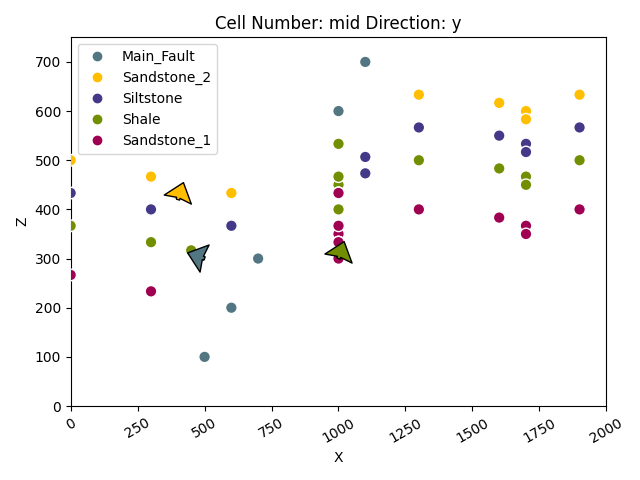Using plot_data_3D, we can also visualize this data in 3D. Note that direct 3D visualization in GemPy requires the Visualization Toolkit (VTK) to be installed.

All 3D plots in GemPy are interactive. This means that we can drag and drop any data point and measurement. The perpendicular axis views in VTK are particularly useful to move points solely on a desired 2D plane. Any changes will then be stored permanently in the “InputData” dataframe. If we want to reset our data points, we will then need to reload our original input data.

Executing the cell below will open a new window with a 3D interactive plot of our data.

gpv = gp.plot_3d(geo_model, image=False, plotter_type='basic')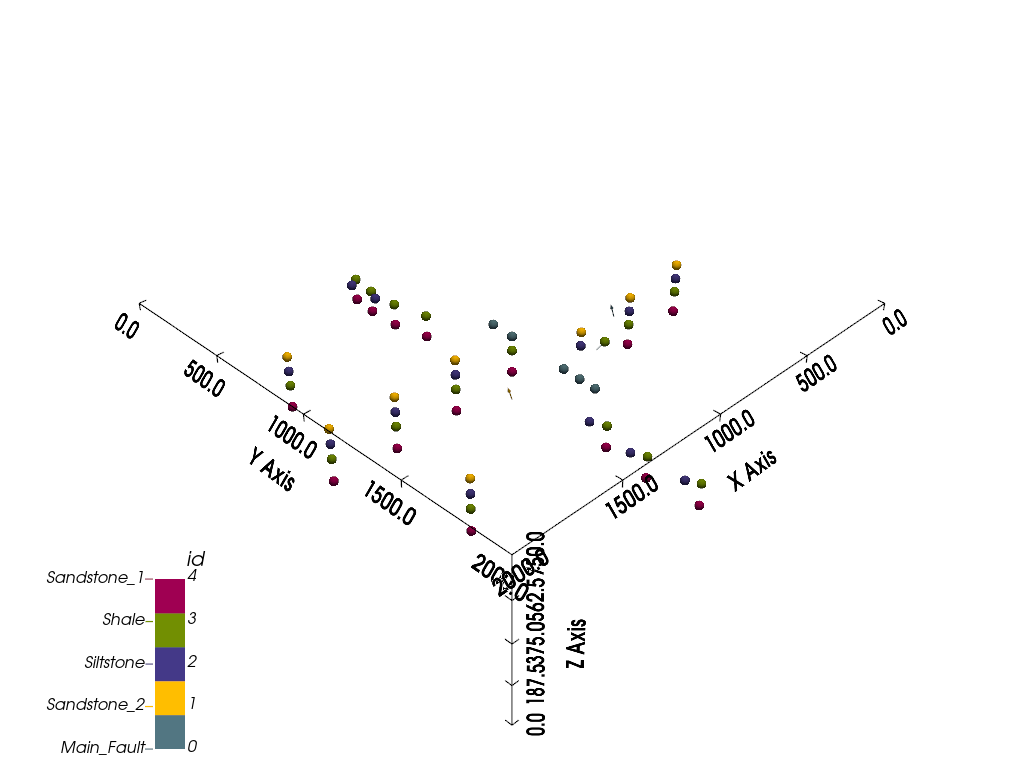### Model generation¶

Once we have made sure that we have defined all our primary information as desired in our object DataManagement.InputData (named geo_data in these tutorials), we can continue with the next step towards creating our geological model: preparing the input data for interpolation.

This is done by generating an InterpolatorData object (named interp_data in these tutorials) from our InputData object via the following function:

gp.set_interpolator(geo_model,
compile_theano=True,
theano_optimizer='fast_compile',
)


Out:

Setting kriging parameters to their default values.
Compiling theano function...
Level of Optimization:  fast_compile
Device:  cpu
Precision:  float64
Number of faults:  1
Compilation Done!
Kriging values:
values
range              2926.17
$C_o$            203869.05
drift equations     [3, 3]

<gempy.core.interpolator.InterpolatorModel object at 0x7fcb8ab2c5e0>


This function rescales the extent and coordinates of the original data (and store it in the attribute geo_data_res which behaves as a usual InputData object) and adds mathematical parameters that are needed for conducting the interpolation. The computation of this step may take a while, as it also compiles a theano function which is required for the model computation. However, should this not be needed, we can skip it by declaring compile_theano = False in the function.

Furthermore, this preparation process includes an assignment of numbers to each formation. Note that GemPy’s always creates a default basement formation as the last formation number. Afterwards, numbers are allocated from youngest to oldest as defined by the sequence of series and formations. On the property formations on our interpolation data, we can find out which number has been assigned to which formation:

The parameters used for the interpolation can be returned using the function get_kriging_parameters. These are generated automatically from the original data, but can be changed if needed. However, users should be careful doing so, if they do not fully understand their significance.

gp.get_data(geo_model, 'kriging')

values
range 2926.17
$C_o$ 203869.05
drift equations [3, 3]

At this point, we have all we need to compute our full model via compute_model. By default, this will return two separate solutions in the form of arrays. The first gives information on the lithological formations, the second on the fault network in the model. These arrays consist of two subarrays as entries each:

1. Lithology block model solution:

• Entry : This array shows what kind of lithological formation is found in each voxel, as indicated by a respective formation_number.

• Entry : Potential field array that represents the orientation of lithological units and layers in the block model.

2. Fault network block model solution:

• Entry : Array in which all fault-separated areas of the model are represented by a distinct number contained in each voxel.

• Entry : Potential field array related to the fault network in the block model.

Below, we illustrate these different model solutions and how they can be used.

sol = gp.compute_model(geo_model)

sol


Out:

Lithology ids
[6. 6. 6. ... 2. 2. 2.]

geo_model.solutions


Out:

Lithology ids
[6. 6. 6. ... 2. 2. 2.]


### Direct model visualization in GemPy¶

Model solutions can be easily visualized in 2D sections in GemPy directly. Let’s take a look at our lithology block:

gp.plot_2d(geo_model, show_data=True)
plt.show()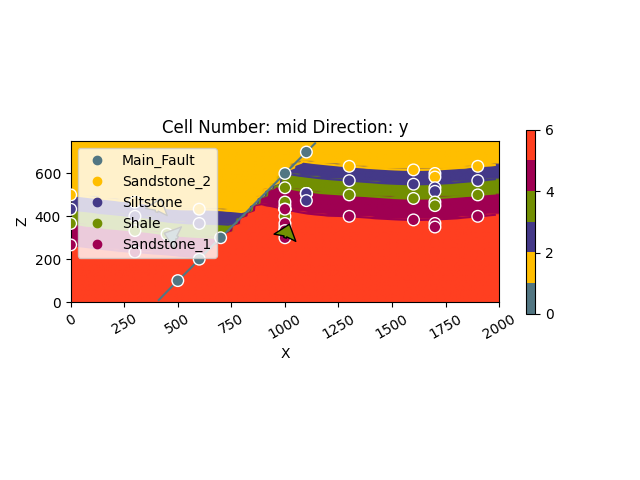With cell_number=25 and remembering that we defined our resolution to be 50 cells in each direction, we have chosen a section going through the middle of our block. We have moved 25 cells in direction='y', the plot thus depicts a plane parallel to the $$x$$- and $$y$$-axes. Setting plot_data=True, we could plot original data together with the results. Changing the values for cell_number and direction, we can move through our 3D block model and explore it by looking at different 2D planes.

We can do the same with out lithological scalar-field solution:

gp.plot_2d(geo_model, show_data=False, show_scalar=True, show_lith=False)
plt.show()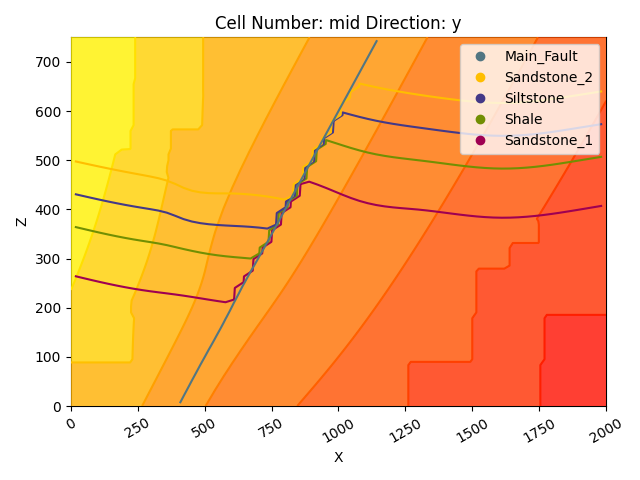gp.plot_2d(geo_model, series_n=1, show_data=False, show_scalar=True, show_lith=False)
plt.show()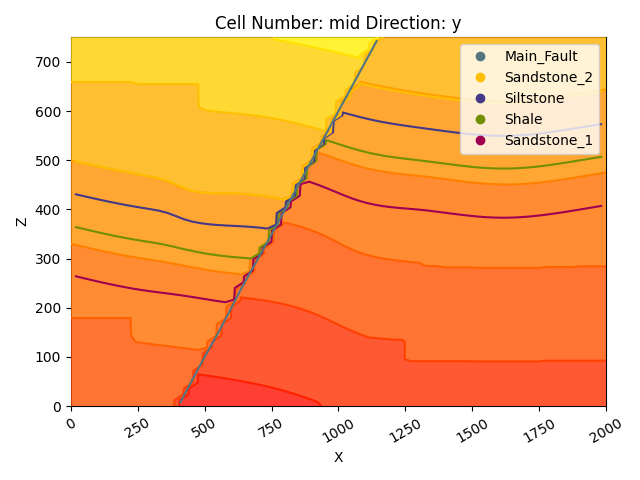This illustrates well the fold-related deformation of the stratigraphy, as well as the way the layers are influenced by the fault.

The fault network modeling solutions can be visualized in the same way:

geo_model.solutions.scalar_field_at_surface_points


Out:

array([[0.03075848, 0.        , 0.        , 0.        , 0.        ],
[0.        , 0.77174354, 0.72471042, 0.80357372, 0.83598092]])

gp.plot_2d(geo_model, show_block=True, show_lith=False)
plt.show()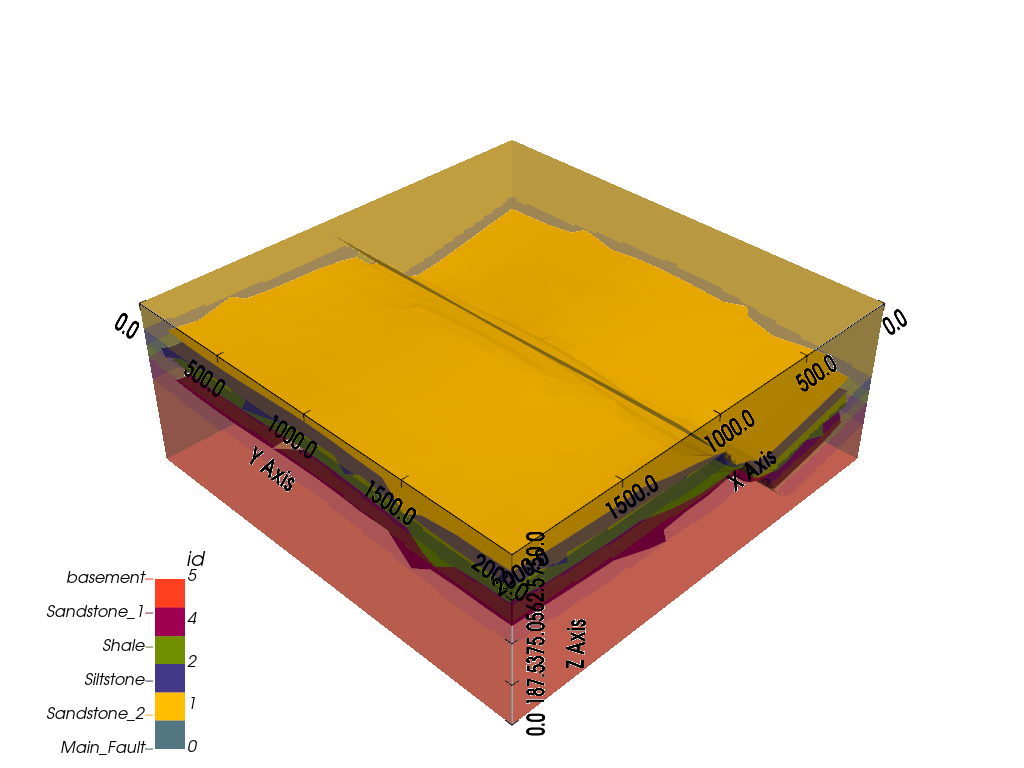gp.plot_2d(geo_model, series_n=1, show_block=True, show_lith=False)
plt.show()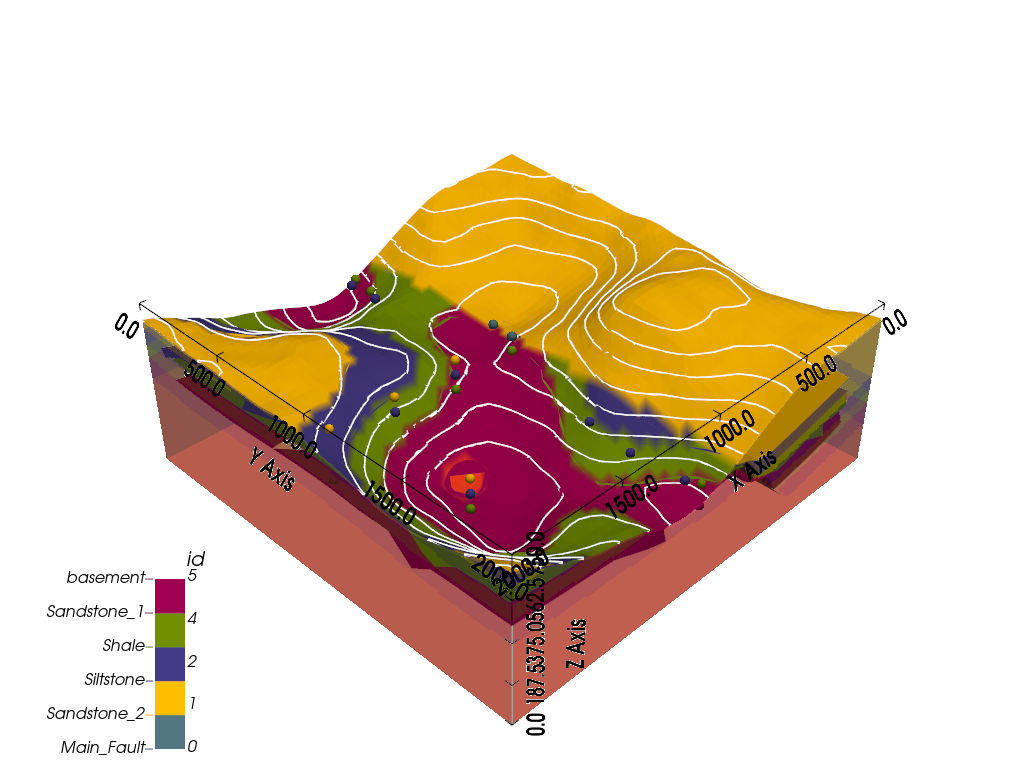### Marching cubes and vtk visualization¶

In addition to 2D sections we can extract surfaces to visualize in 3D renderers. Surfaces can be visualized as 3D triangle complexes in VTK (see function plot_surfaces_3D below). To create these triangles, we need to extract respective vertices and simplices from the potential fields of lithologies and faults. This process is automatized in GemPy with the function get_surface.

ver, sim = gp.get_surfaces(geo_model)
gpv = gp.plot_3d(geo_model, image=False, plotter_type='basic')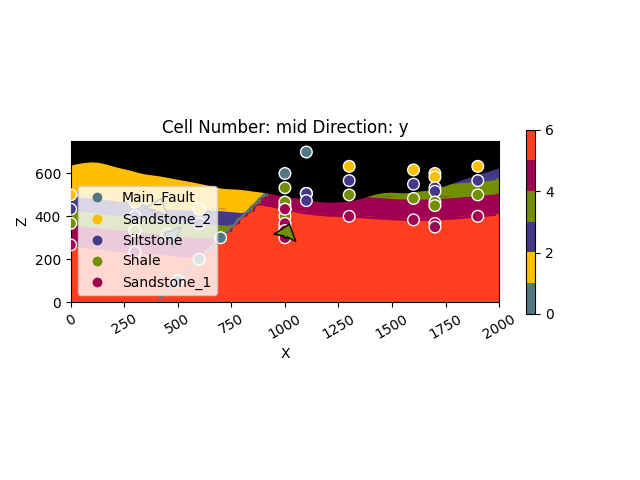Using the rescaled interpolation data, we can also run our 3D VTK visualization in an interactive mode which allows us to alter and update our model in real time. Similarly to the interactive 3D visualization of our input data, the changes are permanently saved (in the InterpolationInput.dataframe object). Additionally, the resulting changes in the geological models are re-computed in real time.

geo_model.set_topography(d_z=(350, 750))


Out:

Active grids: ['regular' 'topography']

Grid Object. Values:
array([[  20.        ,   20.        ,    7.5       ],
[  20.        ,   20.        ,   22.5       ],
[  20.        ,   20.        ,   37.5       ],
...,
[2000.        , 1918.36734694,  423.48951452],
[2000.        , 1959.18367347,  430.25455308],
[2000.        , 2000.        ,  431.07163663]])

gp.compute_model(geo_model)
gp.plot_2d(geo_model, show_topography=True)
plt.show()

# sphinx_gallery_thumbnail_number = 9
gpv = gp.plot_3d(geo_model, plotter_type='basic', show_topography=True, show_surfaces=True,
show_lith=True,
image=False)Out:

/WorkSSD/PythonProjects/gempy/gempy/core/solution.py:173: VisibleDeprecationWarning: Creating an ndarray from ragged nested sequences (which is a list-or-tuple of lists-or-tuples-or ndarrays with different lengths or shapes) is deprecated. If you meant to do this, you must specify 'dtype=object' when creating the ndarray.
self.geological_map = np.array(


### Compute at a given location¶

This is done by modifying the grid to a custom grid and recomputing. Notice that the results are given as grid + surfaces_points_ref + surface_points_rest locations

x_i = np.array([[3, 5, 6]])
sol = gp.compute_model(geo_model, at=x_i)


Out:

Active grids: ['custom']
/WorkSSD/PythonProjects/gempy/gempy/core/solution.py:168: VisibleDeprecationWarning: Creating an ndarray from ragged nested sequences (which is a list-or-tuple of lists-or-tuples-or ndarrays with different lengths or shapes) is deprecated. If you meant to do this, you must specify 'dtype=object' when creating the ndarray.
self.custom = np.array(


Therefore if we just want the value at x_i:

sol.custom


Out:

array([array([[6.]]), array([[0.18630133],
[0.63163565]])], dtype=object)


This return the id, and the scalar field values for each series

### Save the model¶

GemPy uses Python [pickle] for fast storing temporary objects (https://docs.python.org/3/library/pickle.html). However, module version consistency is required. For loading a pickle into GemPy, you have to make sure that you are using the same version of pickle and dependent modules (e.g.: Pandas, NumPy) as were used when the data was originally stored.

For long term-safer storage we can export the pandas.DataFrames to csv by using:

gp.save_model(geo_model)


Out:

True


Total running time of the script: ( 0 minutes 11.939 seconds)

Gallery generated by Sphinx-Gallery